﻿ xCAT - examples Icom

xCAT   EncoderCAT  configuration examples

All the example are for an IC7000   ... which is my only ICOM radio !!

I quote from the IC7000 instruction manual.   You can download this from Icom.

The data presentation is the same for the more modern radios  ... with different  command code allocations.

All configurations have rig address = 0x70  (IC7000 default)  and encat address = 0xE0 (any value should be ok)

NR level

Manual page 146:   command 1A    sub command 050114

Send/read NR level set (0 = 0 to 15 = 15)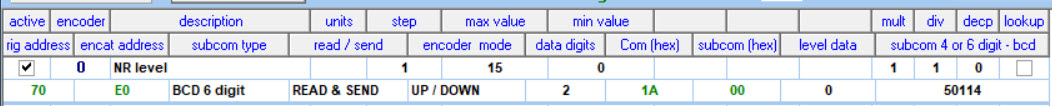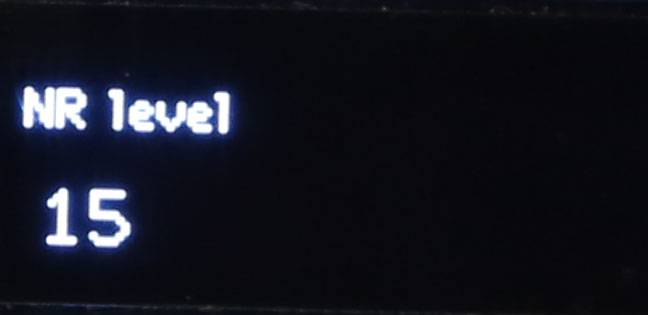Encoder 0   min = 0   max = 15   step = 1   mult and div = 1 (ie no scaling)    decp = 0  (no decimal point)     no lookup command = 0x1A    subcommand(hex) = 0   - this is not used with BCD 6 digit     (the sub command is subcom 4 or 6 digit  = 50114 which codes as 0x05  0x01   0x14) subcom type = BCD 6 digit   read /send = READ & SEND   encoder mode  = UP / DOWN

data digits = 2  (0-15 needs 2 decimal digits  > one bcd byte).   level data unused

NR on / off

Manual page 143:   command 16     sub command 40
Noise reduction (0 = OFF;  1 = ON)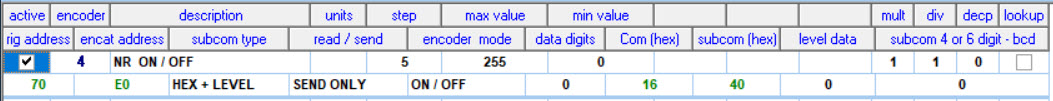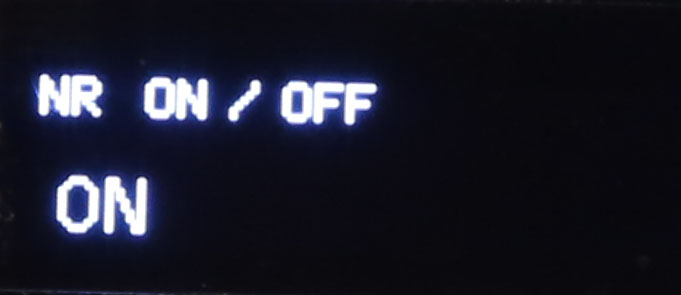Encoder 4     min,   max,  step  and  data digits unused     mult, div and decp  are OLED display controls  -  unused.   OLED displays 0 / 1 as ON / OFF. Command = 16     subcommand (hex) = 40      level data unused   subcom 4 or 6 digit unused subcom type = HEX + LEVEL     read / send - READ ONLY      encoder mode = UP / DOWN

data digits = 0 (unused)     level data = 0  (unused)

RTTY shift width

Manual page 144:   command = 1A   subcommand = 050011

Send /read RTTY shift width   (0 = 170 Hz,  1 = 200 Hz,   2 = 425 Hz.)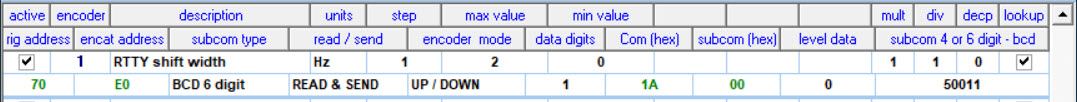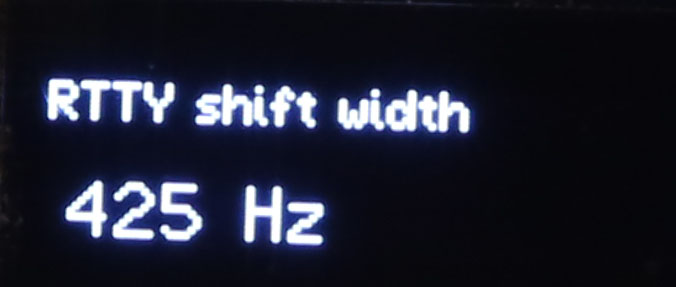Encoder 1   min = 0    max  = 2     step = 1    div = 1   mult = 1 (no scaling)   decp = 0 (unused)    units = Hz Lookup ticked                    See  lookup table settings   >>>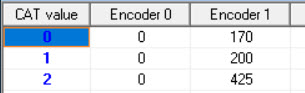command = 0x1A   subcommand (hex) = 0  (unused).   Subcommand is 6 digit decimal: 050011 which codes as 0x05  0x01  0x11

subcom type = BCD 6 digit   read /send = READ & SEND   encoder mode  = UP / DOWN

data digits = 1  (0- 3 needs 1 decimal digits  > one bcd byte).   level data unused

Swap VFO A / B (toggle)

Manual page 142:     command = 0x07   subcommand = 0xB0

Exchange VFO A and VFO B.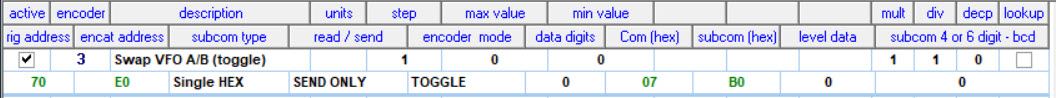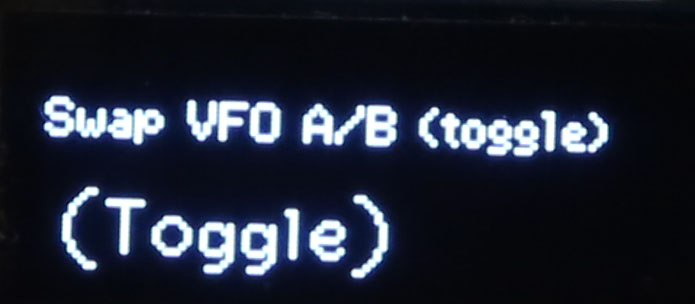Encoder 3    min, max, step, mult, div, decp, lookup  all unusedcommand = 0x07    subcommand (hex) = 0x0B     sub command 4 or 6 digit unused     level data and data digits unused subcom type = single hex    read / send = SEND ONLY     encoder mode = TOGGLE data digits = 0  (unused ).   level data unused

VOX delay

Manual page 146:    command = 1A   subcommand = 050117

Send / read VOX delay (0 = 0.0 sec. to 20 = 29 sec.)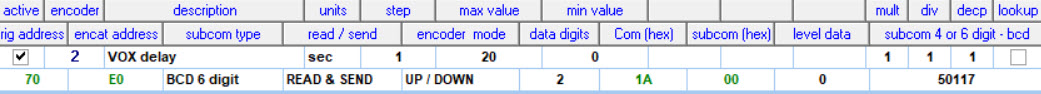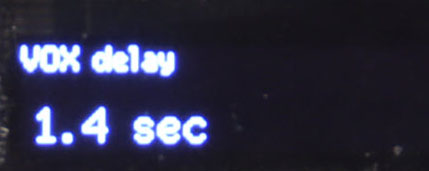Encoder 2   min = 0  max = 20  step = 1   decp = 1 to present in seconds  Units = 'sec'. command = 0x1A   subcoman (hex) = 0 = unused. - this is not used with BCD 6 digit     The sub command is subcom 4 or 6 digit  = 501147which codes as 0x05  0x01   0x17. subcom type = BCD 6 digit   read /send = READ & SEND   encoder mode  = UP / DOWN

data digits = 2  (0-20 needs 2 decimal digits  > one bcd byte).   level data unused

Speech comp level

Manual page 145        command = 1A   subcommand = 050094

Send /read speech compressor level (0 = 0 to 10 = 10)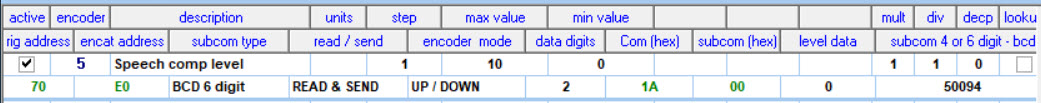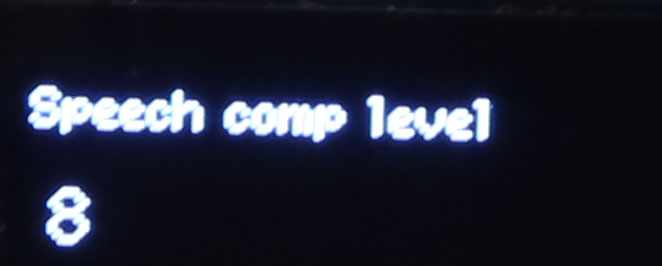Encoder 5   min = 0  max = 10  step = 1   decp = 0 = no dec point. .  Units not set. command = 0x1A   subcom (hex) = 0 = unused. - this is not used with BCD 64 or  digit     The sub command is subcom 4 or 6 digit  = 50094 which codes as 0x05  0x00   0x94. subcom type = BCD 6 digit   read /send = READ & SEND   encoder mode  = UP / DOWN data digits = 2  (0-10 needs 2 decimal digits  > one bcd byte).   level data unused

Frequency 03 > 05

Manual page 142:    command = 03 read  -- EncoderCAT changes to command = 05 to write back.    no subcommand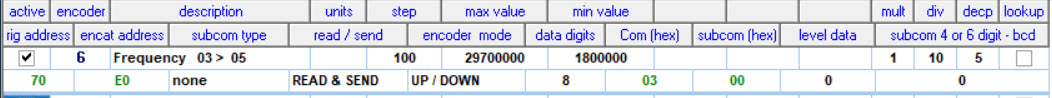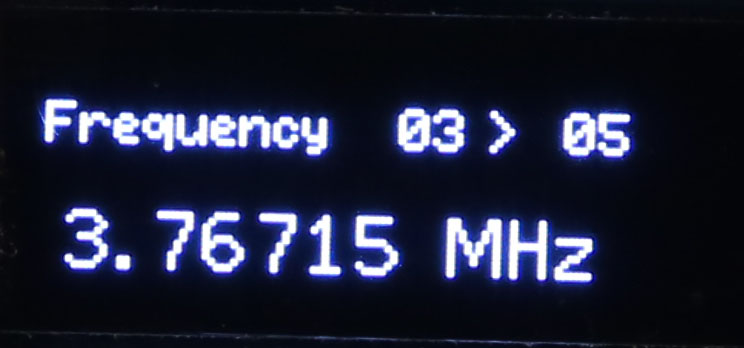Encoder 6    min = 1800000, max = 29700000, step = 100   mult, div  unused      decp = 6  to present in MHz , eg 3.345100   units = MHzcommand = 0x03    no subcommand    EncoderCAT changes command to 0x05 on send back sub command 4 or 6 digit unused     level data and data digits unused subcom type = none    read / send = READ & SEND     encoder mode = UP / DOWN data digits = 0  (unused ).   level data unused

RF power 25% / 100%  switching

... to illustrate binary switching between two preset levels
Manual page 143:    command = 14   subcommand = 0A + Level data

RF power setting ( 0 = mini. to255 = max.)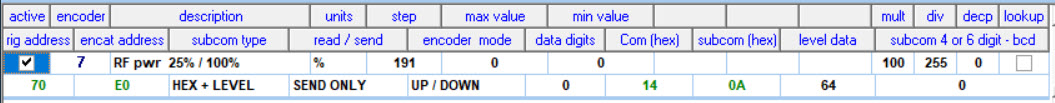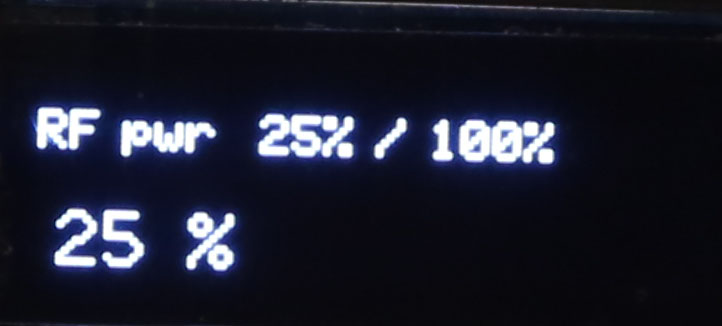Encoder 7   min, max unused   step = 191   level data = 64     Power value switches between 64  on left rotation and  64+191 on right rotation Units = '%'     decp = 0 (no decimal point) command = 0x14    subcom (hex) = 0x0A    subcom 4 or 6 digit  unused    no lookup For OLE display, mult=100 and div=255  scale: value x 100 / 255 to display 0 - 100w

RF power full control range

RF power 0 - 100w

Manual page 143       command = 1A  subcommand = 050001

RF power setting (0 = mini. to 255 = max.)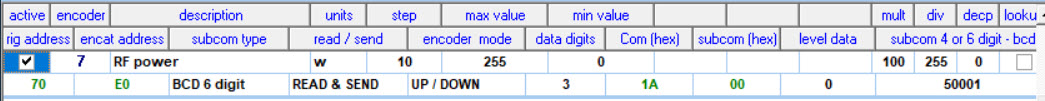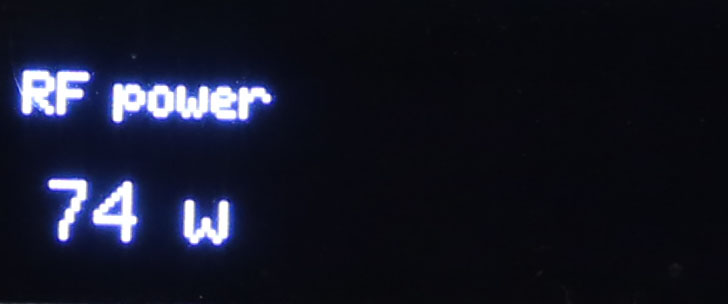Encoder 7   min = 0  max = 255  step = 10   decp = 0 = no dec point. .  Units = 'w' command = 0x1A   subcom (hex) = 0 = unused. - this is not used with BCD 6 or 4 digit     The sub command is subcom 4 or 6 digit  = 50001 which codes as 0x05  0x00   0x01. subcom type = BCD 6 digit   read /send = READ & SEND   encoder mode  = UP / DOWN data digits = 3  (0-255  needs 3 decimal digits  > two bcd bytes).   level data unused For OLE display, mult=100 and div=255  scale: value x 100 / 255 to display 0 - 100w